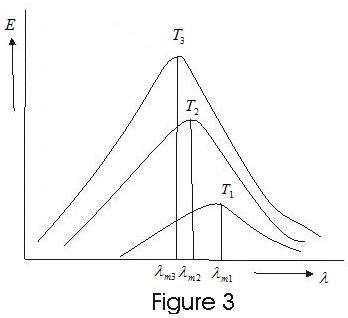# Heat Transfer

Related Topics Assignments Revision Notes

## 7. Stefan Boltzmann law

• The rate urad at which an object emits energy via EM radiation depends on objects surface area A
and temperature T in kelvin of that area and is given by
Where
σ= 5.6703×10-8 W/m2K4
is stefan boltzmann constant and ε is emissivity of object's sunface with value between 0 and 1.
• Black - Body radiator has emissivity of 1.0 which is an ideal limit and does not occuer in nature.
• The rate uabs at which an object absorbs energy via thremal radiation from its environment with temperature Tenv (in kelvin) is
uabs = σεA(Tenv)4               (3)
Where ε is same as in equation 2
• Since an object radiate energy to the enviornment and absorbe energy from environment its net energy enchange due to thermal radiation is
= σεA{(Tenv)4-T4}
(4)
• u is positive if net energy is being absorbed via radiation and negative if it is being lost via radiation.

## 8. Nature of thermal Radiation

• Radiation emitted by a black body is a mixture of waves of different wavelengths and only a small range of wanelength has significant countribution in the total radiation.
• A body is heated at different temperature and Enegy of radiation is plotted agains wavelength is plotted for different temperature we get following curves.• These curves show
(i) Energy is not uniformly distributed in the radiation spectreum of black body.
(ii) At a given temperature the intensity of radiations increases with increase in wavelength, becoms maximum at particular wavelength and further increase in wavelngth leads to decrease in intensity of heat radiation.
(iii) Increase in temperature causes increase in energy emission for all wavelengths.
(iv) Increase in temperature causes decrease in λm, where λm is wavelenght corresponding to highest intersity. This wavelength λm is inversily properational to the absolute temperature of the emitter.
λmT = b                    (5)
Where b is a constant and this equation is known as Wein's displacement law.
b=0.2896×10-2 mk for black body and is known as Wien's constant.